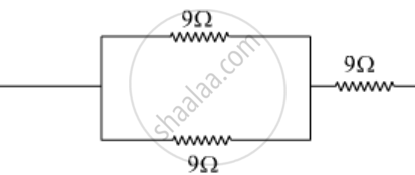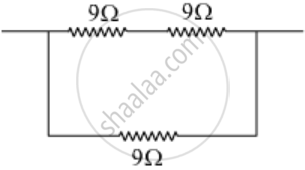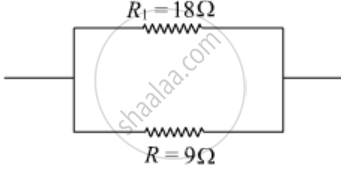Share

# Show How Would You Join Three Resistors, Each of Resistance 9 ω So that the Equivalent Resistance of the Combination Is 1) 13.5 2) 6 ω - CBSE Class 10 - Science

ConceptResistance of a System of Resistors - Resistors in Parallel

#### Question

Show how would you join three resistors, each of resistance 9 Ω so that the equivalent resistance of the combination is

1) 13.5

2) 6 Ω

#### Solution

1) Case: To get equivalent resistance = 13.5 Ω

For this case, two 9 Ω resistors should be first connected in parallel and then their combination should be connected in series with the remaining 9 Ω resistor. Thus,R = (9xx9)/(9+9) = 81/18 = 4.5 ΩR_"total" = R + 9 = 4.5 + 9 = 13.5 Ω

2) Case: To get equivalent resistance = 6 Ω

For this case, two 9 Ω resistors should be first connected in series and then this combination should be connected in parallel with the remaining 9 Ω resistor. Thus,R1 18 ΩR_"total" = (R_1xxR)/(R_1+R) = (18xx9)/(18+9) = 6 Ω

Is there an error in this question or solution?

#### Video TutorialsVIEW ALL 

Solution Show How Would You Join Three Resistors, Each of Resistance 9 ω So that the Equivalent Resistance of the Combination Is 1) 13.5 2) 6 ω Concept: Resistance of a System of Resistors - Resistors in Parallel.
S